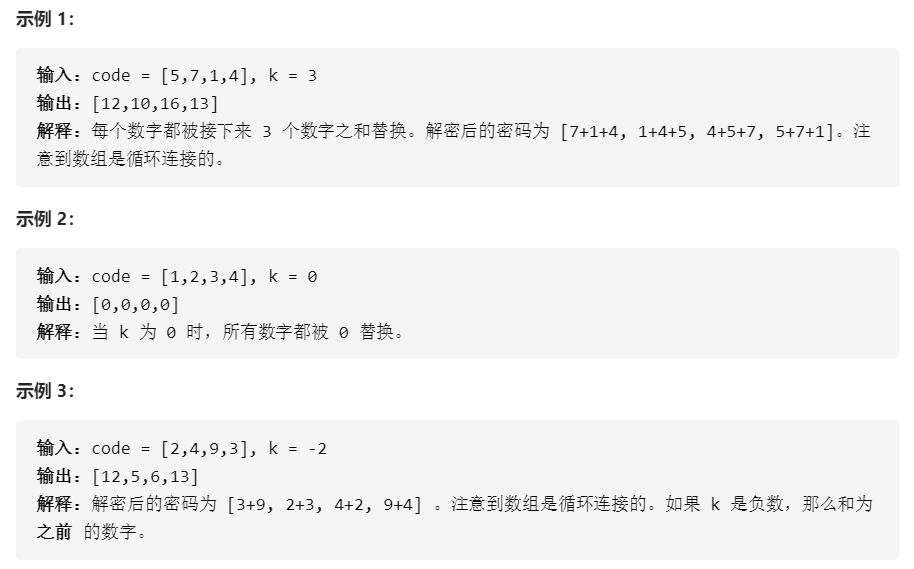2020-12-07 题目类型：简单1298-范同学

2020-12-07 题目类型：简单

1class Solution(object):
def maximumWealth(self, accounts):
"""
:type accounts: List[List[int]]
:rtype: int
"""
m = len(accounts) - 1
result = 0
while m >= 0:
n = len(accounts[m])
temp = 0
for i in range(n):
temp += accounts[m][i]
if temp > result:
result = temp
m -= 1
return result
class Solution:
def maximumWealth(self, accounts):
return max([sum(w) for w in accounts])  # 记住这些函数len，sum，max

2class Solution(object):
def decrypt(self, code, k):
"""
:type code: List[int]
:type k: int
:rtype: List[int]
"""
n = len(code)
if k == 0: return  * n  # 记忆点1号
sign = 1 if k > 0 else -1
res =  * n
for i in range(n):
for j in range(1, abs(k) + 1):  # 记忆点2号abs是绝对值
res[i] += code[(n + i + j * sign) % n]
return res

评论 抢沙发觉得文章有用就打赏一下文章作者

支付宝扫一扫打赏微信扫一扫打赏Vieu3.3主题Q Q 登 录